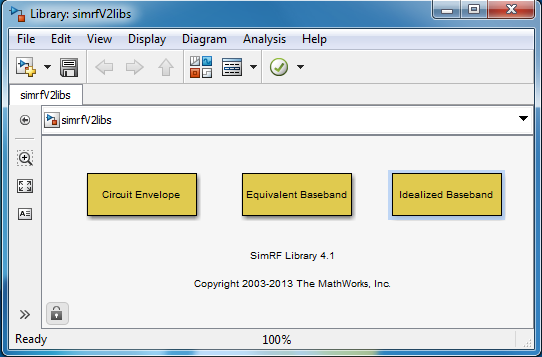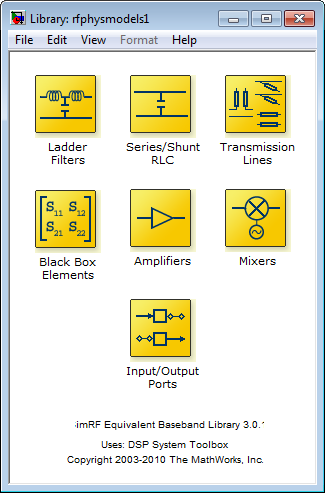## RF Blockset Equivalent Baseband Libraries

### Overview of RF Blockset Equivalent Baseband Libraries

The RF Blockset™ Equivalent Baseband libraries consist of the Equivalent Baseband (physical) and Idealized Baseband (mathematical) libraries of components for modeling RF systems within the Simulink® environment. An RF model can contain blocks from both the physical and mathematical libraries. It can also include Simulink blocks and blocks from other blocksets.

RF Blockset Equivalent Baseband software extends your Simulink modeling environment with a library of blocks for modeling RF systems that include RF filters, transmission lines, amplifiers, and mixers.

You use RF Blockset Equivalent Baseband library blocks to represent the components of your RF system in a Simulink model. The blockset provides several types of component representations using network parameters (S, Y, Z, ABCD, H, and T format), mathematical descriptions, and physical properties.

In the Simulink model, you cascade the components to represent your RF architecture and run the simulation. During the simulation, the model computes a time-domain, complex-baseband representation. This method results in fast simulation of the quadrature modeling schemes used in modern communication systems and enables compatibility with other Simulink blocks.

The blocks let you visualize their specified network parameters using plots and Smith® Charts.

A validated Simulink model of an RF system can provide an executable specification for RF circuit design for wireless communication systems.

You can also use the blockset with Simulink Coder™ software to generate embeddable C code for real-time execution.

### Open RF Blockset Equivalent Baseband Libraries

To open the main library window, type the following at the MATLAB® prompt:

`rflib`

Each yellow icon in the window represents a library. Double-click an icon to open the corresponding library.For a discussion of the Equivalent and Idealized Baseband libraries, see the following sections.

Note

The blue icons take you to the MATLAB Help browser.

• Double-click the Demos icon to open the RF Blockset Equivalent Baseband examples.

• Double-click the Info icon to open the RF Blockset Equivalent Baseband documentation.

### Equivalent Baseband Library

Use blocks from the Equivalent Baseband library to model physical and electrical components by specifying physical properties or by importing measured data. This library includes several sublibraries, as shown in the following figure.The following table describes the sublibraries and how to use them.

SublibraryDescription
AmplifiersRF amplifiers, specified using network parameters, noise data, and nonlinearity data, or a data file containing these parameters.
Ladder FiltersRF filters, specified using LC parameters. The software calculates the network parameters and noise data of the blocks from the topology of the filter and the LC values.
Series/Shunt RLCSeries and shunt RLC components for designing lumped element cascades, specified using RLC parameters. The software calculates the network parameters and noise data of the blocks from the topology of the components and the RLC values.
MixersRF mixers that contain local oscillators, specified using network parameters, noise data, and nonlinearity data, or a data file containing these parameters.
Transmission LinesRF filters, specified using physical dimensions and electrical characteristics. The software calculates the network parameters and noise data of the blocks from the specified data.
Black BoxPassive RF components, specified using network parameters, or a data file containing these parameters. The software calculates the network parameters and noise data of the blocks from the specified data.
Input/Output PortsBlocks for specifying simulation information that pertains to all blocks in a physical subsystem, such as center frequency and sample time. A physical subsystem is a collection of one or more physical blocks bracketed by an Input Port block and an Output Port block that bridge the physical and mathematical parts of the model.

For more information on defining components, see Specify or Import Component Data.

### Idealized Baseband Library

The Idealized Baseband library contains mathematical representations of the amplifier, mixer, and filter blocks. Use a block from this library to model an RF component in terms of mathematical equations that describe how the block operates on an input signal.

Idealized Baseband blocks assume perfect impedance matching and a nominal impedance of 1 ohm. This means there is no loading and the power flow is unidirectional. As such, they are similar to standard Simulink blocks. In contrast, the Equivalent Baseband blocks do not assume perfect matching—these blocks model the reflections that occur between blocks. Physical blocks model bidirectional power flow, and include loading effects. For these blocks, you can specify the source and load impedances using the Input Port and Output Port blocks.

The mathematical library is shown in the following figure.